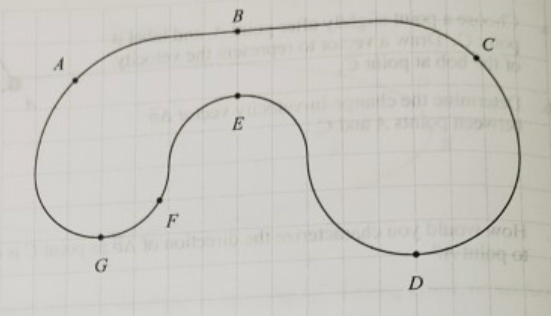# Problem: A car travels clockwise once around the track shown below. Starting from rest at point A, the car speeds up at a constant rate until it is just past point C. By the time it reaches point D, it is traveling at a constant speed. It then travels at a constant speed the rest of the way around the track.a. On the diagram at right, indicate the direction of the acceleration at each of the points A-G. If it is possible to indicate the exact direction of the acceleration, do so, and write “E” for exact. Otherwise, indicate the approximate direction of the acceleration. If the magnitude of the acceleration is zero at any point(s), state so explicitly.b. Is the magnitude of the acceleration at point G greater than, less than, or equal to that at point D? Explain.

###### FREE Expert Solution

a.

The motion between A and C has a constant tangential and radial acceleration.

C to D only has radial acceleration.

91% (321 ratings)###### Problem Details

A car travels clockwise once around the track shown below. Starting from rest at point A, the car speeds up at a constant rate until it is just past point C. By the time it reaches point D, it is traveling at a constant speed. It then travels at a constant speed the rest of the way around the track.

a. On the diagram at right, indicate the direction of the acceleration at each of the points A-G. If it is possible to indicate the exact direction of the acceleration, do so, and write “E” for exact. Otherwise, indicate the approximate direction of the acceleration. If the magnitude of the acceleration is zero at any point(s), state so explicitly.b. Is the magnitude of the acceleration at point G greater than, less than, or equal to that at point D? Explain.